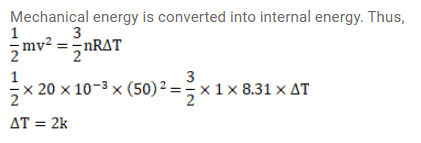# A vessel containing one mole of a monatomic ideal gasQuestion:

A vessel containing one mole of a monatomic ideal gas (molecular weight $=20 \mathrm{~g} / \mathrm{mol}$ ) is moving on a floor at a speed of $50 \mathrm{~m} / \mathrm{s}$. The vessel is stopped suddenly. Assuming that the mechanical energy lost has gone into the internal energy of the gas, find the rise in its temperature.

Solution: